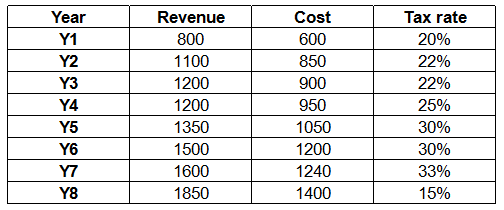# 2017 SSC CGL 08 Aug Shift-3

Instructions

For the following questions answer them individually

Question 91

Question 92

Question 93

Question 94

Question 95

Question 96

# If sin 5θ + sin θ = sin 3θ and 0 < θ < (π/2), then what is the value of θ (in degrees)?

Instructions

The table given below represents the cost, revenue and tax rate for XYZ Limited for a period of 8 years. Cost and revenue are given in Rs '000 crores.Profit for any year = revenue — cost

Profit after tax for any year = profit of that year - tax of that year

tax on any year = tax rate of that year x profit of that year

Question 97

Question 98

Question 99

Question 100

OR